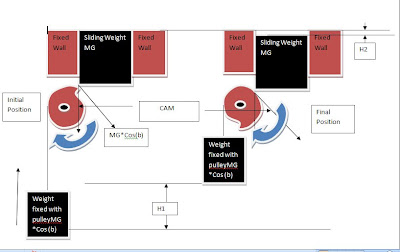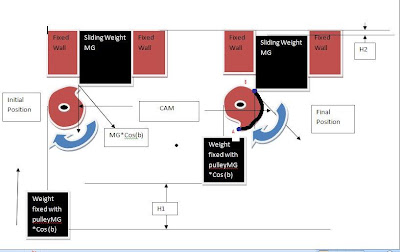## Tuesday, September 8, 2009

### Cam Follower Mechanism Energy Efficiency

You can see cam follower mechanism for clean energy cheaper than coal. My cam follower mechanism is different than today’s cam follower. In general cam follower system,

cam shaft is rotated and follower move up and down according to cam profile.
In my cam follower system,
1. Follower is offset.
2. Force is applied from follower to cam and by using this force cam is rotated.
3. We can decide how much follower come down for helping cam to rotate particular circumference length.
4. Follower down 6 cm with 100 kg and rotate cam of 27 cm at 70 kg.
5. Input energy we use to move follower is =0.06*100 =6 N-Meter
6 Out put energy we get on cam = 0.27*70 = 18.9 N-Meter
You can go through full post to get exact idea.

You can see one cam and two follower. Force is applied by follower on cam. Follower come down 6 cm and help cam to rotate 27 cm peripheral length at little less force(70% of force applied on follower.) Please read the full post to know exactly the benefit of this system and how it works.

I have removed cylinder. I use rubber ring on follower bearing which is in contact with cam. So noise and friction is reduced. My cam follower Mechanism is different then normal cam follower. In general cam follower cam shaft is rotated and according to cam profile follower move up and down. In my cam follower mechanism,
1. Follower is off set. Follower is not inline with cam center.
2. Force is applied from follower to cam. Follower is offset, So when you apply force from         follower to cam, this force help cam to rotate.
3. Follower come down little bit and help cam to rotate more circumference length.
In short follower come down 5.5cm length with 100 kg and rotate cam 27 cm circumference length with 70 kg force.

So we use energy to move follower and get on cam. We get 3 times more energy on cam than we use to move follower.

You can see practical model of cam follower mechanism for clean energy. Follower move little bit and help cam to rotate 6 times more peripheral length. Cam can rotate 360 degree using two followers. Basic thing is follower move little bit and help to rotate cam 6 times more length at little less force(70% of force applied by follower). Please read the full post to know exactly the benefit of this system and how it works.

Model is about using cam for energy generation. You can see green arrow. That is force applied. Force is applied on cam using follower. Follower move up little bit and help to rotate cam at much more peripheral length.

You can see two video attached. You will get the idea.

When you apply force on follower, it will help cam to rotate. Follower move up little bit and help cam to rotate lot more peripheral length. We use the energy to move follower. And we get the energy by rotation of cam.

You can see green arrow in the video, that is force applied.

Question is what is the benefit of this system?

We get more energy on cam than we use to move follower.

We can decide how much follower move up for particular peripheral length of cam.

I mean follower move up only 1cm for helping cam to rotate 10cm peripheral length.

Force applied by follower will divide in two parts. One is tangential and second is towards the center of cam. Tangential part will help cam to rotate.

If we consider pressure angle 45`, you will get 70% of total force applied in tangential direction of cam.

So total energy loss by follower= force * distance

=100*0.01 (we consider force 100 N and distance 1cm (0.01 mtr))

=1N-mtr

Energy got by rotation of cam=Force *distance

=70*0.10 (we get 70% of total force in tangential direction so it is 70 and distance is 10 cm so it is 0.1meter)

=7N-mtr

If you use correctly, your production cost will be cut dramatically.

Model is about using cam for energy generation. force is applied on cam using follower. You can see green aerrow, that is force applied. Follower come down little bit and help to rotate cam at much more Peripheral length.Click on figure for large view. It is about using Cam for increase energy efficiency. Cam are generally used to open and close valve of IC Engine. I am going to show you another use. Left side initial position. Right side final position. Redius of cam continuously decrease.Sliding weight (MG) applied on Cam. With the help of rope weight(MG*Cos(b)) is fixed with cam. We consider b as 45. So cos 45=.70. This fixed weight should be 0.70 MG. Which balance the sliding weight applied on cam in tengential direction. As sliding weight come down the fixed weight go up. But the twist is the redius of cam decrease nominal continuously. So sliding weight come down little bit and fixed weight go up much more. We consider sliding weight come dowm H2 height and fixed weight go up H1 height. We consider H1=5H2
Now we calculate energy loss by sliding weight=MG*Height
=MG*H2
Energy got by lifting fixed weight =MG*Cos(b)*Height
=MG*Cos45*H1
=.70MG*5H2 (Because H1=5H2)
=3.5MGH2
=3.5* Energy loss by sliding weight
So we get 3.5 times more energy than we loss. The ratio depend on the rate we decrease redius of cam and angle (b).-->
The Exact value of H1 is the length of curve from point A to point B. You can easily see black curve on cam from point A (Blue dot ) to point B(Blue dot).
The basic thing is weight (MG) comes down nominal height (H2) and help
to lift little less weight (0.70MG) at much higher height (H1=5H2)

I have explained that we can utilized the gravitational force to
rotate cam. I show that potential energy (mgh) we loss when sliding
weight come down is much less than potential energy we get by lifting
fixed weight. Fixed weight is lifted at equal height of peripheral
distance between starting and final position. It is H1. We reduce
radius of cam marginal so the sliding weight come down nominal . it
should be H2. Sliding weight (MG) applied in vertical downward
direction. When it applied on cam, it will be divided in two parts.
One is tangential ( MG cos (b)). and second is towards the center (MG
sin (b)). Tangential force will help to rotate cam and towards the
center force will generate friction.
The potential energy loss by sliding weight
= mgh
= MG* H2
The potential energy got by lifting fixed weight
=mgh
= MGcos(b)* H1
=MGcos(b)*5H2 (H1=5H2)
=cos(b)*5*MGH2 (b=45, cos(b)=0.70)
=0.70*5* MGH2
= 3.5 * energy loss by sliding plate.
H1=5H2 or may be H1=6H2 or H1=4H2
The ratio depend on the rate we decrease radius of cam. If the base
diameter is 50 mm , we consider two situation.
1. fall is 3mm through 180degree. (fall for cam design)
2. fall is 6 mm through 180 degree. (fall for cam design)
In first case H2=3mm
In second case H2=6 mm
In both case H1 is nearly same.
Click here to view model on you tube.